# Scatter, Box, and Violin Plots

lessR provides many versions of a scatter plot with its `Plot()` function, all accessible with the same simple syntax. Illustrate with the Employee data included as part of lessR.

``d <- Read("Employee")``
``````##
## >>> Suggestions
##
## Data Types
## ------------------------------------------------------------
## character: Non-numeric data values
## integer: Numeric data values, integers only
## double: Numeric data values with decimal digits
## ------------------------------------------------------------
##
##     Variable                  Missing  Unique
##         Name     Type  Values  Values  Values   First and last values
## ------------------------------------------------------------------------------------------
##  1     Years   integer     36       1      16   7  NA  15 ... 1  2  10
##  2    Gender character     37       0       2   M  M  M ... F  F  M
##  3      Dept character     36       1       5   ADMN  SALE  SALE ... MKTG  SALE  FINC
##  4    Salary    double     37       0      37   53788.26  94494.58 ... 56508.32  57562.36
##  5    JobSat character     35       2       3   med  low  low ... high  low  high
##  6      Plan   integer     37       0       3   1  1  3 ... 2  2  1
##  7       Pre   integer     37       0      27   82  62  96 ... 83  59  80
##  8      Post   integer     37       0      22   92  74  97 ... 90  71  87
## ------------------------------------------------------------------------------------------``````

As an option, also read the table of variable labels. Create the table formatted as two columns. The first column is the variable name and the second column is the corresponding variable label. Not all variables need to be entered into the table. The table can be a `csv` file or an Excel file.

Currently, read the label file into the l data frame. The labels are displayed on both the text and visualization output. Each displayed label consists of the variable name juxtaposed with the corresponding label.

``l <- rd("Employee_lbl")``
``````##
## >>> Suggestions
##
## Data Types
## ------------------------------------------------------------
## character: Non-numeric data values
## ------------------------------------------------------------
##
##     Variable                  Missing  Unique
##         Name     Type  Values  Values  Values   First and last values
## ------------------------------------------------------------------------------------------
##  1     label character      8       0       8   Time of Company Employment ... Test score on legal issues after instruction
## ------------------------------------------------------------------------------------------``````

## Continuous Variables

### Two Variables

The typical scatterplot visualizes the relationship of two continuous variables, here Years worked at a company, and annual Salary. Following is the function call to `Plot()` for the default visualization. Because d is the default name of the data frame that contains the variables for analysis, the `data` parameter that names the input data frame need not be specified.

``Plot(Years, Salary)``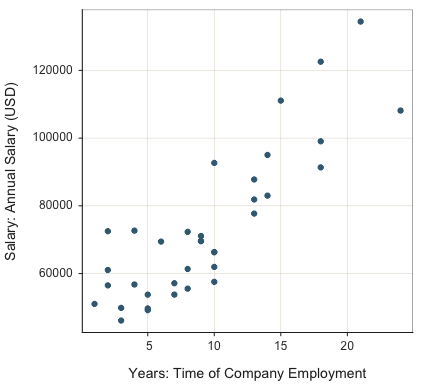``````## >>> Suggestions
## Plot(Years, Salary, fit="lm", fit_se=c(.90,.99))  # fit line, standard errors
## Plot(Years, Salary, out_cut=.10)  # label top 10% potential outliers
## Plot(Years, Salary, enhance=TRUE)  # many options
##
##
## >>> Pearson's product-moment correlation
##
## Years: Time of Company Employment
## Salary: Annual Salary (USD)
##
## Number of paired values with neither missing, n = 36
##
##
## Sample Correlation of Years and Salary: r = 0.852
##
##
## Hypothesis Test of 0 Correlation:  t = 9.501,  df = 34,  p-value = 0.000
## 95% Confidence Interval for Correlation:  0.727 to 0.923``````

Enhance the default scatterplot with parameter `enhance`. The visualization includes the mean of each variable indicated by the respective line through the scatterplot, the 95% confidence ellipse, labeled outliers, least-squares regression line with 95% confidence interval, and the corresponding regression line with the outliers removed.

``Plot(Years, Salary, enhance=TRUE)``
``## [Ellipse with Murdoch and Chow's function ellipse from their ellipse package]``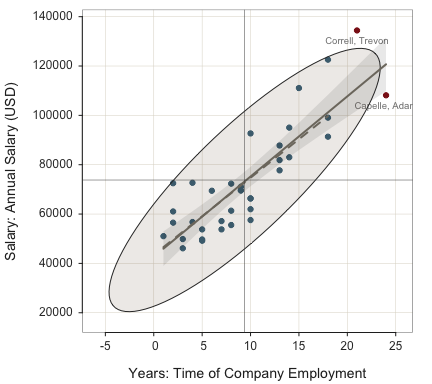``````## >>> Suggestions
## Plot(Years, Salary, fit="lm", fit_se=c(.90,.99))  # fit line, standard errors
## Plot(Years, Salary, out_cut=.10)  # label top 10% potential outliers
##
##
## >>> Pearson's product-moment correlation
##
## Years: Time of Company Employment
## Salary: Annual Salary (USD)
##
## Number of paired values with neither missing, n = 36
##
##
## Sample Correlation of Years and Salary: r = 0.852
##
##
## Hypothesis Test of 0 Correlation:  t = 9.501,  df = 34,  p-value = 0.000
## 95% Confidence Interval for Correlation:  0.727 to 0.923``````
``````## >>> Outlier analysis with Mahalanobis Distance
##
##   MD                  ID
## -----               -----
## 8.14     Correll, Trevon
##
## 5.63  Korhalkar, Jessica
## 5.58       James, Leslie
## 3.75         Hoang, Binh
## ...                 ...``````

A variety of fit lines can be plotted. The available values: `"loess"` for general non-linear fit, `"lm"` for linear least squares, `"null"` for the null (flat line) model, `"exp"` for the exponential model, `"sqrt"` for the square root model, and `"reciprocal"` for the reciprocal model. Setting `fit` to `TRUE` plots the `"loess"` line.

Here, plot general non-linear fit. For emphasis set `plot_errors` to `TRUE` to plot the residuals from the line.

``Plot(Years, Salary, fit="loess", plot_errors=TRUE)``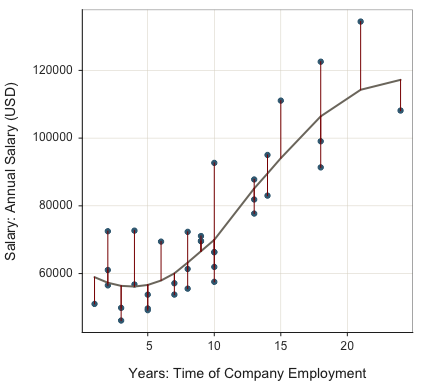``````## >>> Suggestions
## Plot(Years, Salary, out_cut=.10)  # label top 10% potential outliers
## Plot(Years, Salary, enhance=TRUE)  # many options
##
##
## >>> Pearson's product-moment correlation
##
## Years: Time of Company Employment
## Salary: Annual Salary (USD)
##
## Number of paired values with neither missing, n = 36
##
##
## Sample Correlation of Years and Salary: r = 0.852
##
##
## Hypothesis Test of 0 Correlation:  t = 9.501,  df = 34,  p-value = 0.000
## 95% Confidence Interval for Correlation:  0.727 to 0.923``````

Next, plot the exponential fit and show the residuals from the exponential curve. These data are approximately linear so the exponential curve does not vary far fom a straight line.

``Plot(Years, Salary, fit="exp", plot_errors=TRUE)``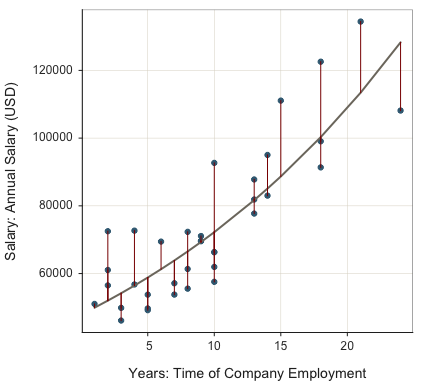``````## >>> Suggestions
## Plot(Years, Salary, out_cut=.10)  # label top 10% potential outliers
## Plot(Years, Salary, enhance=TRUE)  # many options
##
##
## >>> Pearson's product-moment correlation
##
## Years: Time of Company Employment
## Salary: Annual Salary (USD)
##
## Number of paired values with neither missing, n = 36
##
##
## Sample Correlation of Years and Salary: r = 0.852
##
##
## Hypothesis Test of 0 Correlation:  t = 9.501,  df = 34,  p-value = 0.000
## 95% Confidence Interval for Correlation:  0.727 to 0.923``````

### Three Plus Variables

Map a continuous variable, such as Pre, to the plotted points with the `size` parameter, a bubble plot.

``Plot(Years, Salary, size=Pre)``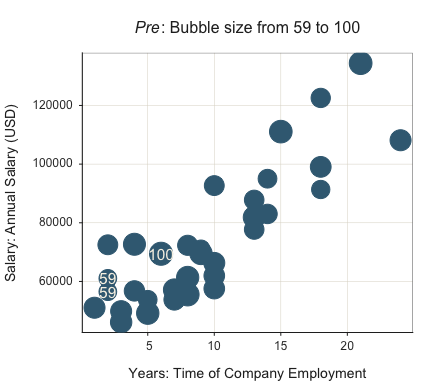``````## >>> Suggestions
## Plot(Years, Salary, fit="lm", fit_se=c(.90,.99))  # fit line, standard errors
## Plot(Years, Salary, out_cut=.10)  # label top 10% potential outliers
## Plot(Years, Salary, enhance=TRUE)  # many options
## Plot(x=Years, y=Salary, size=Pre, radius=0.18) # larger bubbles
##
##
## >>> Pearson's product-moment correlation
##
## Years: Time of Company Employment
## Salary: Annual Salary (USD)
##
## Number of paired values with neither missing, n = 36
##
##
## Sample Correlation of Years and Salary: r = 0.852
##
##
## Hypothesis Test of 0 Correlation:  t = 9.501,  df = 34,  p-value = 0.000
## 95% Confidence Interval for Correlation:  0.727 to 0.923``````
``````##
##
## Some Parameter values (can be manually set)
## -------------------------------------------------------
## fill: #3C6A82   filled color of the points
## color: #3C6A82  edge color of the points
## radius: 0.12        size of largest bubble
## power: 0.50     relative bubble sizes``````

Indicate multiple variables to plot along either axis with a vector defined according to the base R function `c()`. Plot the linear model for each variable according to the `fit` parameter set to `"lm"`. Turn off the confidence interval by setting the standard errors to zero with `fit_se` set to `0`.

``Plot(c(Pre, Post), Salary, fit="lm", fit_se=0)``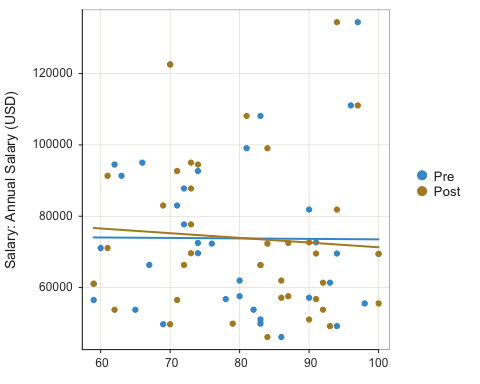``````## >>> Suggestions
## Plot(c(Pre, Post), Salary, out_cut=.10)  # label top 10% potential outliers
## Plot(c(Pre, Post), Salary, enhance=TRUE)  # many options
##
##
## >>> Pearson's product-moment correlation
##
## Pre: Test score on legal issues before instruction
## Salary: Annual Salary (USD)
##
## Number of paired values with neither missing, n = 37
##
##
## Sample Correlation of Pre and Salary: r = -0.007
##
##
## Hypothesis Test of 0 Correlation:  t = -0.043,  df = 35,  p-value = 0.966
## 95% Confidence Interval for Correlation:  -0.330 to 0.318
##
## >>> Pearson's product-moment correlation
##
## Post: Test score on legal issues after instruction
## Salary: Annual Salary (USD)
##
## Number of paired values with neither missing, n = 37
##
##
## Sample Correlation of Post and Salary: r = -0.070
##
##
## Hypothesis Test of 0 Correlation:  t = -0.416,  df = 35,  p-value = 0.680
## 95% Confidence Interval for Correlation:  -0.385 to 0.260``````

### Scatterplot Matrix

Three or more variables for the first parameter value plot as a scatterplot matrix. Pass a single vector, such as defined by `c()`. Request the non-linear fit line and corresponding confidence interval by specifying `TRUE` or `loess` for the `fit` parameter. Request a linear fit line with the value of `"lm"`.

``Plot(c(Salary, Years, Pre, Post), fit=TRUE)``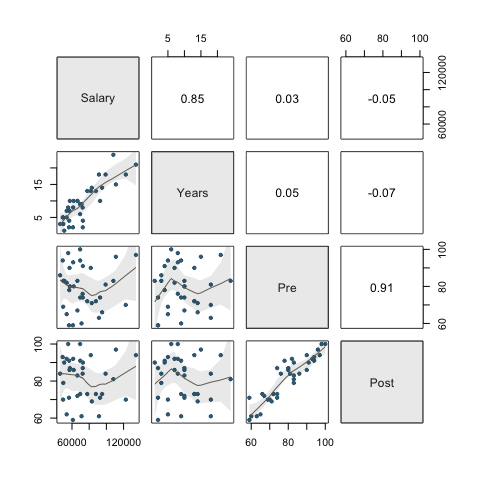### Smoothed Scatterplot

Generate random data with base R `rnorm()`, then plot. `Plot()` first checks the presence of the specified variables in the global environment (workspace). If not there, then from a data frame, of which the default value is d. Here, generate values for x and y in the workspace.

``````x <- rnorm(4000)
y <- rnorm(4000)
Plot(x, y)``````
``````## >>> Note: x is from the workspace, not in a data frame (table)
## >>> Note: y is from the workspace, not in a data frame (table)``````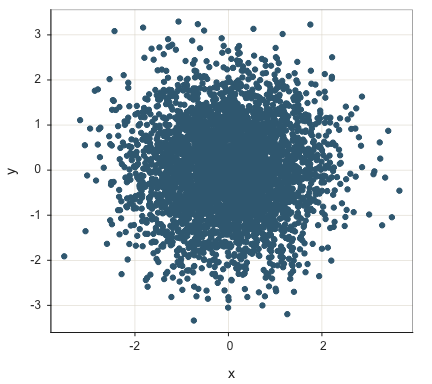``````## >>> Suggestions
## Plot(x, y, fit="lm", fit_se=c(.90,.99))  # fit line, standard errors
## Plot(x, y, out_cut=.10)  # label top 10% potential outliers
## Plot(x, y, enhance=TRUE)  # many options
##
##
## >>> Pearson's product-moment correlation
##
## Number of paired values with neither missing, n = 4000
##
##
## Sample Correlation of x and y: r = 0.006
##
##
## Hypothesis Test of 0 Correlation:  t = 0.390,  df = 3998,  p-value = 0.696
## 95% Confidence Interval for Correlation:  -0.025000000000 to 0.037000000000``````

With large data sets, even for continuous variables there can be much over-plotting of points. One strategy to address this issue smooths the scatterplot. The individual points superimposed on the smoothed plot are potential outliers. The default number plotted is 100. Turn off completely by setting parameter `smooth_points` to `0`.

``Plot(x, y, smooth=TRUE)``
``````## >>> Note: x is from the workspace, not in a data frame (table)
## >>> Note: y is from the workspace, not in a data frame (table)``````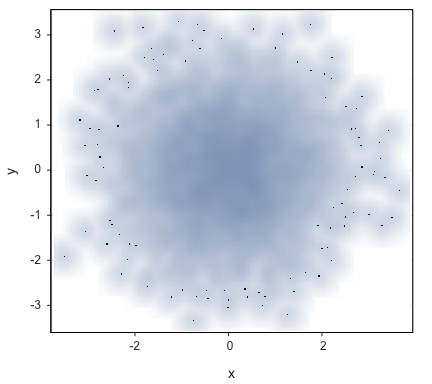``````## >>> Suggestions
## Plot(x, y, fit="lm", fit_se=c(.90,.99))  # fit line, standard errors
## Plot(x, y, out_cut=.10)  # label top 10% potential outliers
## Plot(x, y, enhance=TRUE)  # many options
##
##
## >>> Pearson's product-moment correlation
##
## Number of paired values with neither missing, n = 4000
##
##
## Sample Correlation of x and y: r = 0.006
##
##
## Hypothesis Test of 0 Correlation:  t = 0.390,  df = 3998,  p-value = 0.696
## 95% Confidence Interval for Correlation:  -0.025000000000 to 0.037000000000``````

Another strategy for alleviating over-plotting makes the fill color mostly transparent with the `trans` parameter, or turn off completely by setting to `fill` to `"off"`. The closer the value of `trans` is to 1, the more transparent is the fill.

``Plot(x, y, trans=0.95)``
``````## >>> Note: x is from the workspace, not in a data frame (table)
## >>> Note: y is from the workspace, not in a data frame (table)``````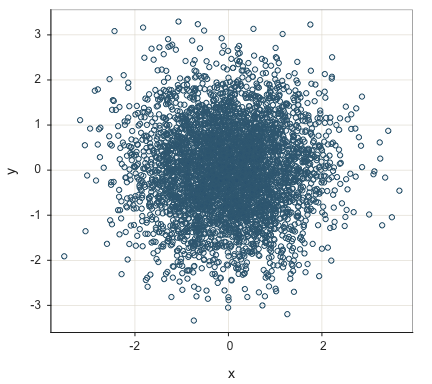``````## >>> Suggestions
## Plot(x, y, fit="lm", fit_se=c(.90,.99))  # fit line, standard errors
## Plot(x, y, out_cut=.10)  # label top 10% potential outliers
## Plot(x, y, enhance=TRUE)  # many options
##
##
## >>> Pearson's product-moment correlation
##
## Number of paired values with neither missing, n = 4000
##
##
## Sample Correlation of x and y: r = 0.006
##
##
## Hypothesis Test of 0 Correlation:  t = 0.390,  df = 3998,  p-value = 0.696
## 95% Confidence Interval for Correlation:  -0.025000000000 to 0.037000000000``````

### One Variable

The default plot for a single continuous variable includes not only the scatterplot, but also the superimposed violin plot and box plot, with outliers identified. Call this plot the VBS plot.

``Plot(Salary)``
``````## [Violin/Box/Scatterplot graphics from Deepayan Sarkar's lattice package]
## >>> Suggestions
## Plot(Salary, out_cut=2, fences=TRUE, vbs_mean=TRUE)  # Label two outliers ...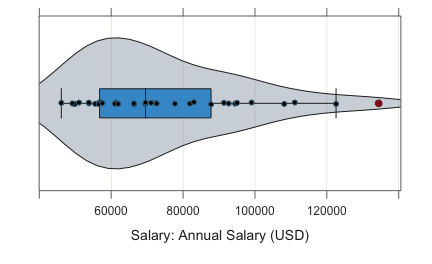``````## --- Salary ---
## Present: 37
## Missing: 0
## Total  : 37
##
## Mean         : 73795.557
## Stnd Dev     : 21799.533
## IQR          : 31012.560
## Skew         : 0.190   [medcouple, -1 to 1]
##
## Minimum      : 46124.970
## Lower Whisker: 46124.970
## 1st Quartile : 56772.950
## Median       : 69547.600
## 3rd Quartile : 87785.510
## Upper Whisker: 122563.380
## Maximum      : 134419.230
##
##
## (Box plot) Outliers: 1
##
## Small      Large
## -----      -----
##            Correll, Trevon 134419.23
##
##
## Number of duplicated values: 0
##
##
## Parameter values (can be manually set)
## -------------------------------------------------------
## size: 0.61      size of plotted points
## out_size: 0.82  size of plotted outlier points
## jitter_y: 0.45 random vertical movement of points
## jitter_x: 0.00  random horizontal movement of points
## bw: 9529.04       set bandwidth higher for smoother edges``````

Control the choice of the three superimposed plots – violin, box, and scatter – with the `vbs_plot` parameter. The default setting is `vbs` for all three plots. Here, for example, obtain just the box plot. Or, use the alias `BoxPlot()` in place of `Plot()`.

``Plot(Salary, vbs_plot="b")``
``````## [Violin/Box/Scatterplot graphics from Deepayan Sarkar's lattice package]
## >>> Suggestions
## Plot(Salary, out_cut=2, fences=TRUE, vbs_mean=TRUE)  # Label two outliers ...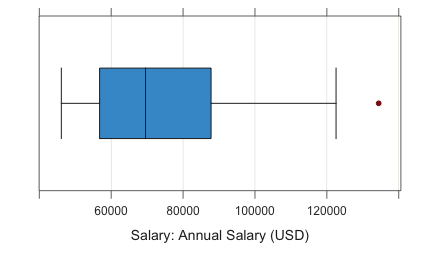``````## --- Salary ---
## Present: 37
## Missing: 0
## Total  : 37
##
## Mean         : 73795.557
## Stnd Dev     : 21799.533
## IQR          : 31012.560
## Skew         : 0.190   [medcouple, -1 to 1]
##
## Minimum      : 46124.970
## Lower Whisker: 46124.970
## 1st Quartile : 56772.950
## Median       : 69547.600
## 3rd Quartile : 87785.510
## Upper Whisker: 122563.380
## Maximum      : 134419.230
##
##
## (Box plot) Outliers: 1
##
## Small      Large
## -----      -----
##            Correll, Trevon 134419.23
##
##
## Number of duplicated values: 0``````

### Cleveland Dot Plot

Create a Cleveland dot plot when one of the variables has unique (ID) values. In this example, for a single variable, row names are on the y-axis. The default plots sorts by the value plotted.

``Plot(Salary, row_names)``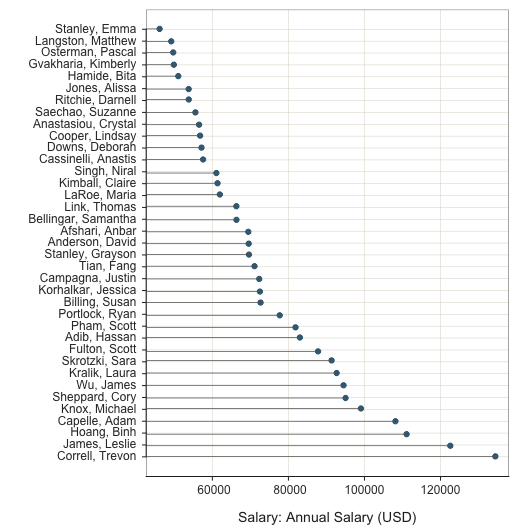``````## >>> Suggestions
## Plot(Salary, y=row_names, sort_yx=FALSE, segments_y=FALSE)
##
##
##
## --- Salary ---
##
##      n   miss      mean        sd       min       mdn       max
##      37      0   73795.6   21799.5   46125.0   69547.6  134419.2
##
##
## (Box plot) Outliers: 1
##
## Small      Large
## -----      -----
##             134419.2``````
``````##
##
## Some Parameter values (can be manually set)
## -------------------------------------------------------
## fill: #3C6A82   filled color of the points
## color: #3C6A82  edge color of the points
## size: 0.80  size of plotted points
## jitter_y: 0.60  random vertical movement of points
## jitter_x: 0.00  random horizontal movement of points``````

The standard scatterplot version of a Cleveland dot plot.

``Plot(Salary, row_names, sort_yx="0", segments_y=FALSE)``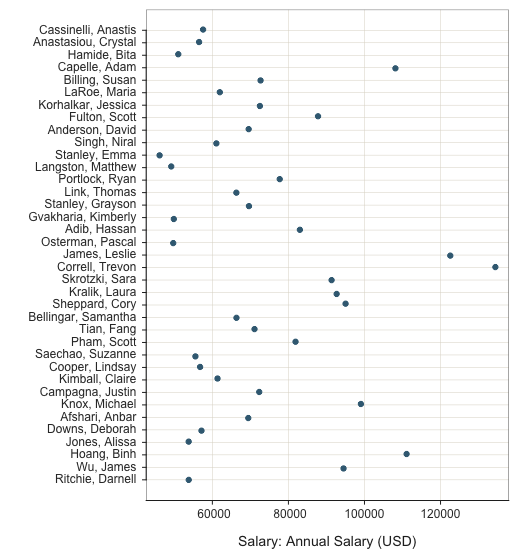``````## >>> Suggestions
##
##
##
## --- Salary ---
##
##      n   miss      mean        sd       min       mdn       max
##      37      0   73795.6   21799.5   46125.0   69547.6  134419.2
##
##
## (Box plot) Outliers: 1
##
## Small      Large
## -----      -----
##             134419.2``````
``````##
##
## Some Parameter values (can be manually set)
## -------------------------------------------------------
## fill: #3C6A82   filled color of the points
## color: #3C6A82  edge color of the points
## size: 0.80  size of plotted points
## jitter_y: 0.60  random vertical movement of points
## jitter_x: 0.00  random horizontal movement of points``````

This Cleveland dot plot has two x-variables, indicated as a standard R vector with the `c()` function. In this situation, the two points on each row are connected with a line segment. By default the rows are sorted by distance between the successive points.

``Plot(c(Pre, Post), row_names)``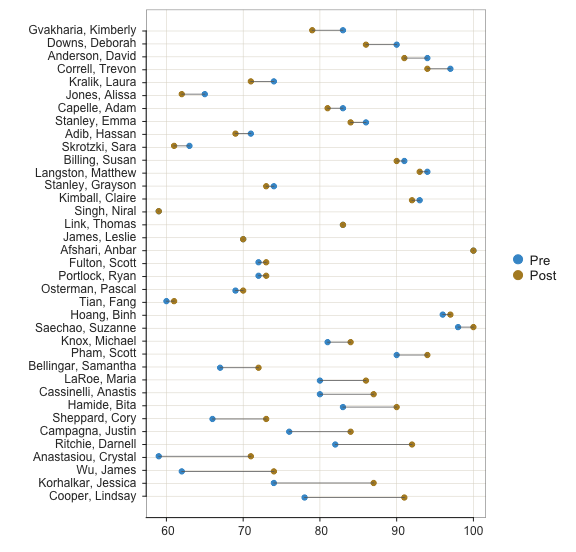``````## >>> Suggestions
## Plot(c(Pre, Post), y=row_names, sort_yx=FALSE, segments_y=FALSE)
##
##
##
## --- Pre ---
##
##      n   miss    mean      sd     min     mdn     max
##      37      0    78.8    12.0    59.0    80.0   100.0
##
##
## --- Post ---
##
##      n   miss    mean      sd     min     mdn     max
##      37      0    81.0    11.6    59.0    84.0   100.0
##
##
## No (Box plot) outliers
##
##
##  n  diff  Row
## ---------------------------
##  1 -4.0 Gvakharia, Kimberly
##  2 -4.0 Downs, Deborah
##  3 -3.0 Anderson, David
##  4 -3.0 Correll, Trevon
##  5 -3.0 Kralik, Laura
##  6 -3.0 Jones, Alissa
##  8 -2.0 Stanley, Emma
## 10 -2.0 Skrotzki, Sara
## 27  5.0 Bellingar, Samantha
## 28  6.0 LaRoe, Maria
## 29  7.0 Cassinelli, Anastis
## 30  7.0 Hamide, Bita
## 31  7.0 Sheppard, Cory
## 32  8.0 Campagna, Justin
## 33 10.0 Ritchie, Darnell
## 34 12.0 Anastasiou, Crystal
## 35 12.0 Wu, James
## 36 13.0 Korhalkar, Jessica
## 37 13.0 Cooper, Lindsay``````
``````##
##
## Some Parameter values (can be manually set)
## -------------------------------------------------------
## fill: #4398D0   filled color of the points
## color: #4398D0  edge color of the points
## size: 0.80  size of plotted points
## jitter_y: 0.60  random vertical movement of points
## jitter_x: 0.00  random horizontal movement of points``````

## Categorical and Continuous Variables

A mixture of categorical and continuous variables can be plotted a variety of ways, as illustrated below.

### Two Continuous, One Categorical

Plot a scatterplot of two continuous variables for each level of a categorical variable on the same panel with the `by` parameter. Here, plot Years and Salary each for the two levels of Gender in the data. Colors and geometric plot shapes can distinguish between the plots. For all variables except an ordered factor, the default plots according to the default qualitative color palette, `"hues"`, with the geometric shape of a point.

``Plot(Years, Salary, by=Gender)``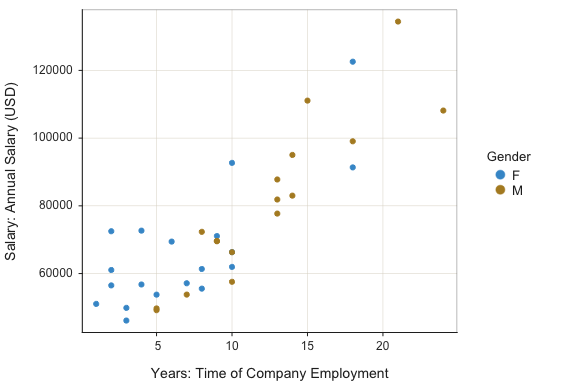``````## >>> Suggestions
## Plot(Years, Salary, fit="lm", fit_se=c(.90,.99))  # fit line, standard errors
## Plot(Years, Salary, out_cut=.10)  # label top 10% potential outliers
## Plot(Years, Salary, enhance=TRUE)  # many options
##
##
## >>> Pearson's product-moment correlation
##
## Years: Time of Company Employment
## Salary: Annual Salary (USD)
##
## Number of paired values with neither missing, n = 36
##
##
## Sample Correlation of Years and Salary: r = 0.852
##
##
## Hypothesis Test of 0 Correlation:  t = 9.501,  df = 34,  p-value = 0.000
## 95% Confidence Interval for Correlation:  0.727 to 0.923``````

If the `by` variable is an ordered factor, the default color palette is sequential according to the underlying theme, such as `"blues"` for the default theme of `"colors"`. Change the general theme with the `style()` function.

``````d\$Gender.f <- factor(d\$Gender, ordered=TRUE)
Plot(Years, Salary, by=Gender.f)``````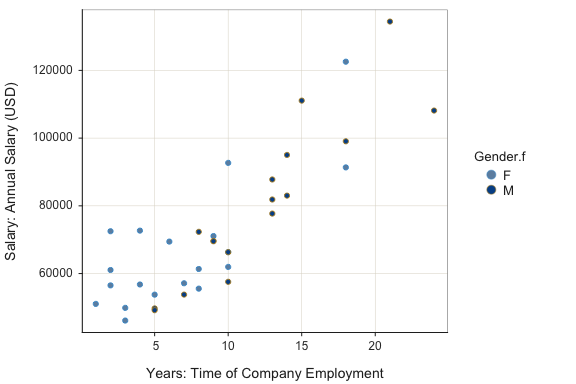``````## >>> Suggestions
## Plot(Years, Salary, fit="lm", fit_se=c(.90,.99))  # fit line, standard errors
## Plot(Years, Salary, out_cut=.10)  # label top 10% potential outliers
## Plot(Years, Salary, enhance=TRUE)  # many options
##
##
## >>> Pearson's product-moment correlation
##
## Years: Time of Company Employment
## Salary: Annual Salary (USD)
##
## Number of paired values with neither missing, n = 36
##
##
## Sample Correlation of Years and Salary: r = 0.852
##
##
## Hypothesis Test of 0 Correlation:  t = 9.501,  df = 34,  p-value = 0.000
## 95% Confidence Interval for Correlation:  0.727 to 0.923``````

Change the plot colors with the `fill` (interior) and `color` (exterior or edge) parameters. Because there are two levels of the `by` variable, specify two fill colors and two edge colors each with an R vector defined by the `c()` function. Also include the regression line for each group and increase the size of the plotted points.

``````Plot(Years, Salary, by=Gender, size=2, fit="lm",
fill=c("olivedrab3", "gold1"),
color=c("darkgreen", "gold4")
)``````